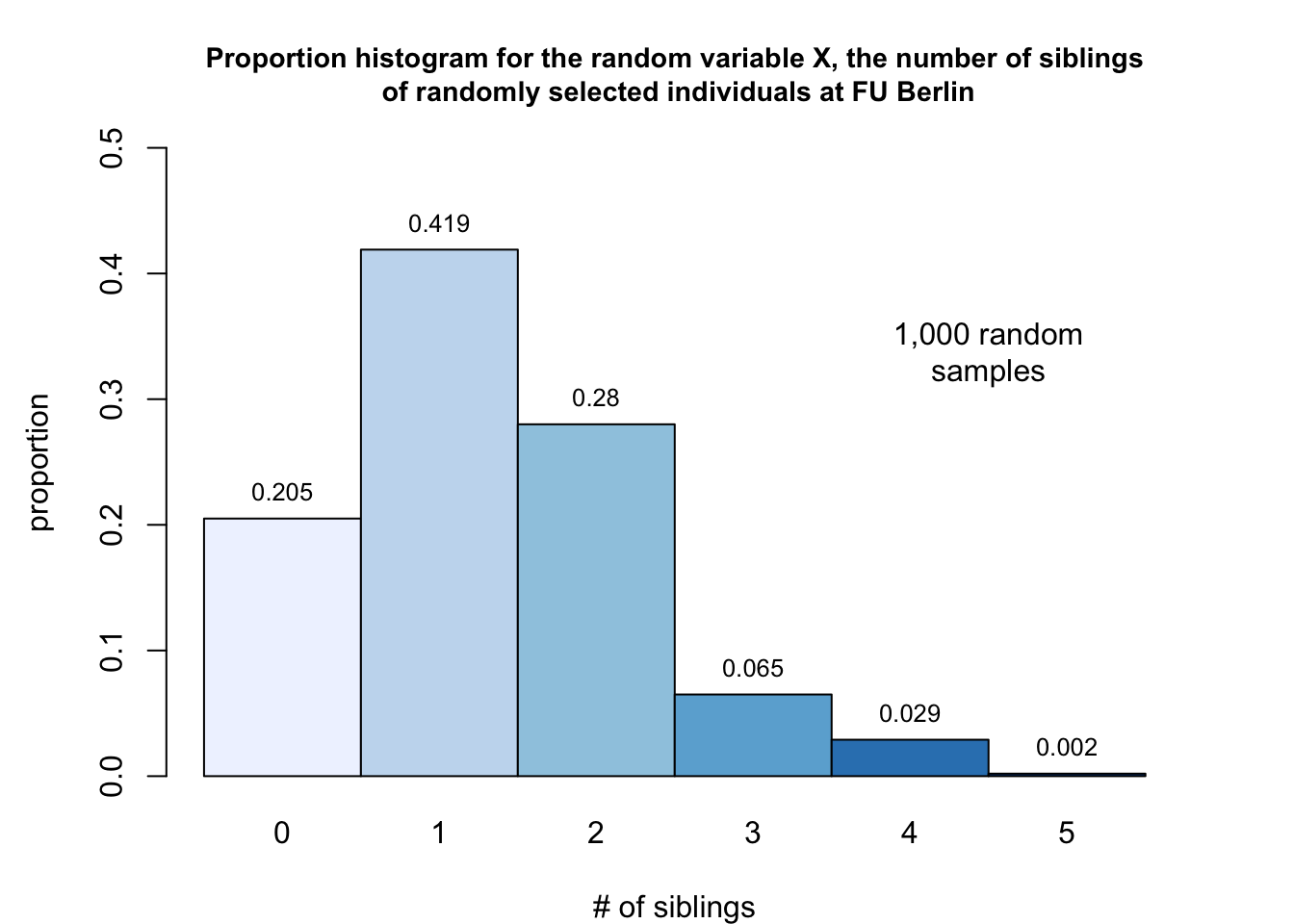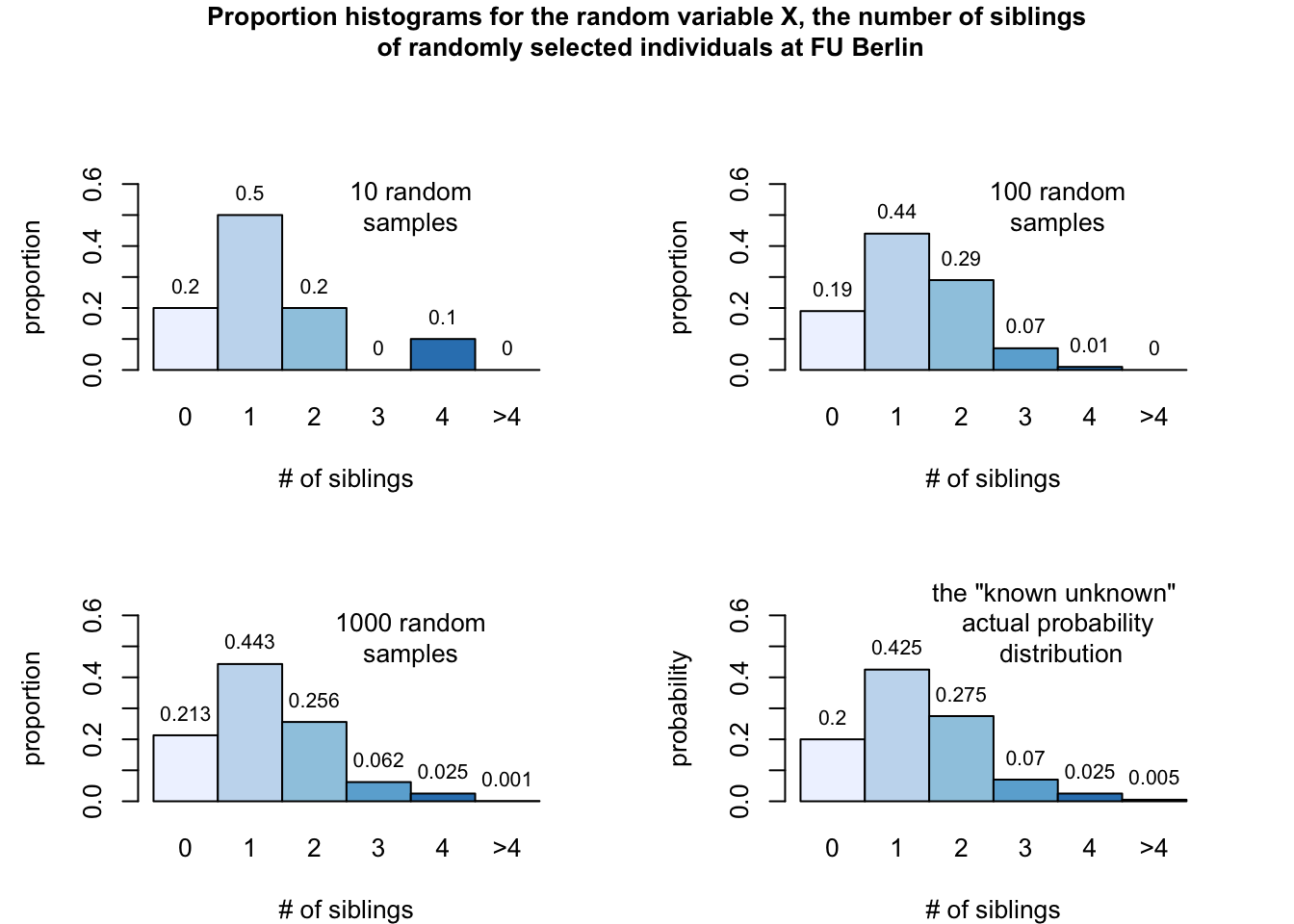Let us elaborate the concept of discrete random variables by an exercise.

Say, our population under investigation consists of all students, all lecturers and all administrative staff members at FU Berlin. We randomly pick on of those individuals and ask him/her about his/her number of siblings. Consequently, the answer, the number of siblings of a randomly selected individual is a discrete random variable, denoted as $$X$$. The actual value (number of siblings) of $$X$$ depends on chance, but we may still list all values of $$X$$, e.g. 0 sibling, 1 sibling, 2 siblings, and so on. For simplification purposes we limit the number of siblings in this exercise to 5.

According the the website of FU Berlin there are 30,600 students, 5,750 doctoral students, 341 professors and 4,270 staff members associated to FU Berlin. In total there are 40,961 individuals (please note, that the actual numbers might change over time) at FU Berlin.

As we do not have any idea of the associated probability for a particular number of sibling, we start some experiments:

We pick one randomly chosen individual and ask for the number of siblings.

The answers are: 4, 0, 2, 0, 2, 2, 1, 2, 0, 3

We pick one hundred individuals and ask for siblings.

The answers are: 2, 0, 1, 2, 2, 0, 0, 0, 1, 3, 1, 2, 1, 0, 2, 0, 0, 2, 1, 1, 1, 1, 2, 2, 1, 2, 2, 0, 1, 1, 2, 4, 0, 3, 2, 0, 1, 2, 2, 2, 1, 2, 1, 1, 2, 1, 2, 2, 2, 1, 1, 1, 1, 1, 1, 2, 1, 1, 2, 2, 1, 1, 0, 2, 0, 1, 0, 1, 2, 1, 2, 1, 2, 2, 2, 1, 0, 2, 2, 4, 1, 2, 1, 1, 1, 1, 1, 0, 2, 1, 0, 1, 0, 1, 1, 2, 0, 2, 0, 0

You see, the form of notation is getting quite fast confusing if we increase the number of individuals we interrogate. Thus, we decide to note the frequency, and the corresponding relative frequency of the values given for the classes 0, 1, 2, 3, 4, 5 (to be explicit: the last class corresponds to 5 or more siblings), and present the experiment in form of a nicely formatted table.

$\begin{array}{c|lcr} \text{Siblings} & \text{Frequency} & \text{Relative}\\ \ x & f & \text{frequency}\\ \hline 0 & 205 & 0.205 \\ 1 & 419 & 0.419 \\ 2 & 280 & 0.28 \\ 3 & 65 & 0.065 \\ 4 & 29 & 0.029 \\ 5 & 2 & 0.002 \\ \hline & 1000 & 1 \end{array}$

After we listed all possible values and calculated the corresponding relative frequencies, we still do not know exactly the probabilities of the discrete random variable $$X$$ for the whole population of 40,961 individuals, associated to FU Berlin. However, after talking to 1,000 randomly chosen individuals we are quite confident that such a large number of interviews - compared to the number of the whole population (40,961) - will give us a good approximation of the probabilities of the discrete random variable $$X$$ (number of siblings) for the whole population.

In a next step, we draw a proportion histogram (of the sample), which displays the possible values of a discrete random variable $$X$$ on the horizontal axis and the proportions of those values on the vertical axis. A proportion histogram may serve as an approximation to the probability distribution too. Please note, that the sum of the probabilities as well as the sum of the proportions of any discrete random variables is 1.In many real life applications we do not know the population’s probability distribution - and we never will. This is mainly because in many applications the population is much to large, or there is no chance to get reliable data, nor we have the money nor the time for exhaustive data collection. However, by increasing the number of independent observations of a random variable $$X$$, the proportion histogram of the sample will approximate better and better the probability histogram of the whole population. To prove this claim we scale up our experiment:

We sequentially pick 10, 100 and 1,000 randomly chosen individuals associated with FU Berlin and ask them about the number of siblings. We will plot each of our three experiments and finally compare it to the actual/real probability distribution (Please note that this example is a toy example and does not represent the real number of sibling in the population of individuals at the FU Berlin; thus the instructors of the present e-learning module know the probability distribution of the population ;-))The graphs confirm our hypothesis, by increasing the number of observations the proportion histogram of the sample will approximate better and better the probability histogram of the whole population.